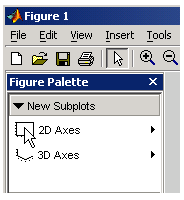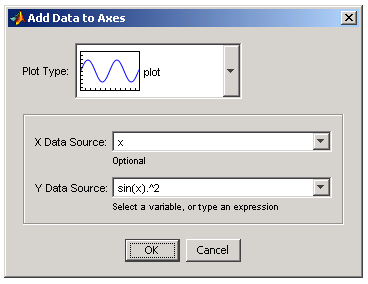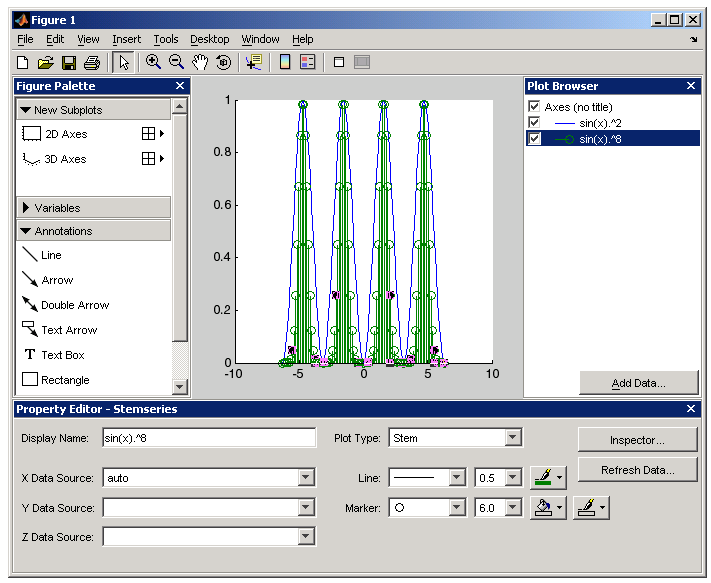GraphicsExample -- Working with Plotting Tools

This example illustrates how to use the plotting tools to graph a workspace variable vs. an expression typed into the Add Data to Axes dialog.

Create a variable in the workspace,

• ```x = -2*pi:pi/25:2*pi;
```

Use the `plottools` command to create a figure with the plotting tools attached.

• ```plottools
```

Click 2D Axes in the New Subplot panel of the Figure Palette.Once the axes appears, the Add Data button on the Plot Browser is activated. Click this button to display the Add Data to Axes dialog.

When the Add Data to Axes dialog is displayed, enter the following values:

• Select plot as the Plot Type.
• Set X Data Source to `x.`
• Set Y Data Source to `sin(x).^2.`
• Click OK to plot this data.MATLAB draws a plot of `sin(x).^2` vs. `x`.

Now add another plot to the same axes. Click Add Data again and specify the data to plot:

• Set X Data Source to `x`.
• Set Y Data Source set to `sin(x).^8`.
• Click OK to plot this data.

Select the last plot (the green line) and set the Plot Type in the Property Editor to `Stem`. The plot should now look like the following picture.Accessing All Object Properties -- Property Inspector Adding a Subplot© 1994-2005 The MathWorks, Inc.